# Wavelet

A wavelet is a wave-like oscillation with an amplitude that begins at zero, increases, and then decreases back to zero. It can typically be visualized as a "brief oscillation" like one recorded by a seismograph or heart monitor. Generally, wavelets are purposefully crafted to have specific properties that make them useful for signal processing. Wavelets can be combined, using a "reverse, shift, multiply and integrate" technique called convolution, with portions of a known signal to extract information from the unknown signal.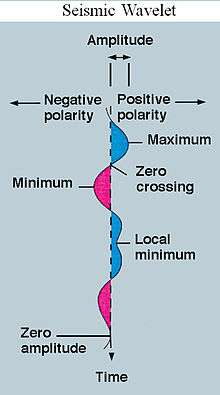Seismic wavelet

For example, a wavelet could be created to have a frequency of Middle C and a short duration of roughly a 32nd note. If this wavelet was to be convolved with a signal created from the recording of a song, then the resulting signal would be useful for determining when the Middle C note was being played in the song. Mathematically, the wavelet will correlate with the signal if the unknown signal contains information of similar frequency. This concept of correlation is at the core of many practical applications of wavelet theory.

As a mathematical tool, wavelets can be used to extract information from many different kinds of data, including – but certainly not limited to – audio signals and images. Sets of wavelets are generally needed to analyze data fully. A set of "complementary" wavelets will decompose data without gaps or overlap so that the decomposition process is mathematically reversible. Thus, sets of complementary wavelets are useful in wavelet based compression/decompression algorithms where it is desirable to recover the original information with minimal loss.

In formal terms, this representation is a wavelet series representation of a square-integrable function with respect to either a complete, orthonormal set of basis functions, or an overcomplete set or frame of a vector space, for the Hilbert space of square integrable functions.

## Name

The word wavelet has been used for decades in digital signal processing and exploration geophysics. The equivalent French word ondelette meaning "small wave" was used by Morlet and Grossmann in the early 1980s.

## Wavelet theory

Wavelet theory is applicable to several subjects. All wavelet transforms may be considered forms of time-frequency representation for continuous-time (analog) signals and so are related to harmonic analysis. Almost all practically useful discrete wavelet transforms use discrete-time filterbanks. These filter banks are called the wavelet and scaling coefficients in wavelets nomenclature. These filterbanks may contain either finite impulse response (FIR) or infinite impulse response (IIR) filters. The wavelets forming a continuous wavelet transform (CWT) are subject to the uncertainty principle of Fourier analysis respective sampling theory: Given a signal with some event in it, one cannot assign simultaneously an exact time and frequency response scale to that event. The product of the uncertainties of time and frequency response scale has a lower bound. Thus, in the scaleogram of a continuous wavelet transform of this signal, such an event marks an entire region in the time-scale plane, instead of just one point. Also, discrete wavelet bases may be considered in the context of other forms of the uncertainty principle.

Wavelet transforms are broadly divided into three classes: continuous, discrete and multiresolution-based.

### Continuous wavelet transforms (continuous shift and scale parameters)

In continuous wavelet transforms, a given signal of finite energy is projected on a continuous family of frequency bands (or similar subspaces of the Lp function space L2(R) ). For instance the signal may be represented on every frequency band of the form [f, 2f] for all positive frequencies f > 0. Then, the original signal can be reconstructed by a suitable integration over all the resulting frequency components.

The frequency bands or subspaces (sub-bands) are scaled versions of a subspace at scale 1. This subspace in turn is in most situations generated by the shifts of one generating function ψ in L2(R), the mother wavelet. For the example of the scale one frequency band [1, 2] this function iswith the (normalized) sinc function. That, Meyer's, and two other examples of mother wavelets are: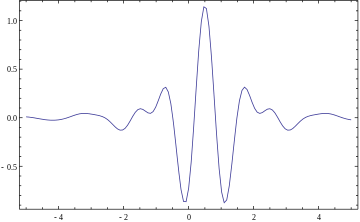Meyer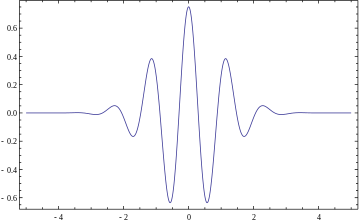Morlet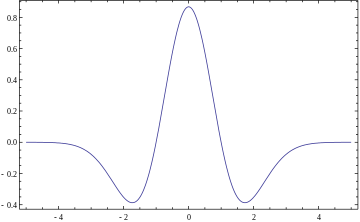Mexican hat

The subspace of scale a or frequency band [1/a, 2/a] is generated by the functions (sometimes called child wavelets)where a is positive and defines the scale and b is any real number and defines the shift. The pair (a, b) defines a point in the right halfplane R+ × R.

The projection of a function x onto the subspace of scale a then has the formwith wavelet coefficientsFor the analysis of the signal x, one can assemble the wavelet coefficients into a scaleogram of the signal.

See a list of some Continuous wavelets.

### Discrete wavelet transforms (discrete shift and scale parameters)

It is computationally impossible to analyze a signal using all wavelet coefficients, so one may wonder if it is sufficient to pick a discrete subset of the upper halfplane to be able to reconstruct a signal from the corresponding wavelet coefficients. One such system is the affine system for some real parameters a > 1, b > 0. The corresponding discrete subset of the halfplane consists of all the points (am, namb) with m, n in Z. The corresponding child wavelets are now given asA sufficient condition for the reconstruction of any signal x of finite energy by the formulais that the functionsform an orthonormal basis of L2(R).

### Multiresolution based discrete wavelet transforms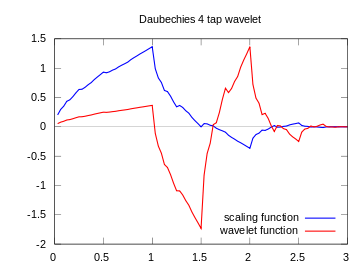D4 wavelet

In any discretised wavelet transform, there are only a finite number of wavelet coefficients for each bounded rectangular region in the upper halfplane. Still, each coefficient requires the evaluation of an integral. In special situations this numerical complexity can be avoided if the scaled and shifted wavelets form a multiresolution analysis. This means that there has to exist an auxiliary function, the father wavelet φ in L2(R), and that a is an integer. A typical choice is a = 2 and b = 1. The most famous pair of father and mother wavelets is the Daubechies 4-tap wavelet. Note that not every orthonormal discrete wavelet basis can be associated to a multiresolution analysis; for example, the Journe wavelet admits no multiresolution analysis.

From the mother and father wavelets one constructs the subspacesThe father waveletkeeps the time domain properties, while the mother waveletskeeps the frequency domain properties.

From these it is required that the sequenceforms a multiresolution analysis of L2 and that the subspacesare the orthogonal "differences" of the above sequence, that is, Wm is the orthogonal complement of Vm inside the subspace Vm−1,In analogy to the sampling theorem one may conclude that the space Vm with sampling distance 2m more or less covers the frequency baseband from 0 to 2m-1. As orthogonal complement, Wm roughly covers the band [2m−1, 2m].

From those inclusions and orthogonality relations, especially, follows the existence of sequencesandthat satisfy the identitiesso thatandso thatThe second identity of the first pair is a refinement equation for the father wavelet φ. Both pairs of identities form the basis for the algorithm of the fast wavelet transform.

From the multiresolution analysis derives the orthogonal decomposition of the space L2 asFor any signal or functionthis gives a representation in basis functions of the corresponding subspaces aswhere the coefficients areand.

## Mother wavelet

For practical applications, and for efficiency reasons, one prefers continuously differentiable functions with compact support as mother (prototype) wavelet (functions). However, to satisfy analytical requirements (in the continuous WT) and in general for theoretical reasons, one chooses the wavelet functions from a subspace of the spaceThis is the space of measurable functions that are absolutely and square integrable:andBeing in this space ensures that one can formulate the conditions of zero mean and square norm one:is the condition for zero mean, andis the condition for square norm one.

For ψ to be a wavelet for the continuous wavelet transform (see there for exact statement), the mother wavelet must satisfy an admissibility criterion (loosely speaking, a kind of half-differentiability) in order to get a stably invertible transform.

For the discrete wavelet transform, one needs at least the condition that the wavelet series is a representation of the identity in the space L2(R). Most constructions of discrete WT make use of the multiresolution analysis, which defines the wavelet by a scaling function. This scaling function itself is a solution to a functional equation.

In most situations it is useful to restrict ψ to be a continuous function with a higher number M of vanishing moments, i.e. for all integer m < MThe mother wavelet is scaled (or dilated) by a factor of a and translated (or shifted) by a factor of b to give (under Morlet's original formulation):For the continuous WT, the pair (a,b) varies over the full half-plane R+ × R; for the discrete WT this pair varies over a discrete subset of it, which is also called affine group.

These functions are often incorrectly referred to as the basis functions of the (continuous) transform. In fact, as in the continuous Fourier transform, there is no basis in the continuous wavelet transform. Time-frequency interpretation uses a subtly different formulation (after Delprat).

Restriction：

(1)when a1 = a and b1 = b,

(2)has a finite time interval

## Comparisons with Fourier transform (continuous-time)

The wavelet transform is often compared with the Fourier transform, in which signals are represented as a sum of sinusoids. In fact, the Fourier transform can be viewed as a special case of the continuous wavelet transform with the choice of the mother wavelet. The main difference in general is that wavelets are localized in both time and frequency whereas the standard Fourier transform is only localized in frequency. The Short-time Fourier transform (STFT) is similar to the wavelet transform, in that it is also time and frequency localized, but there are issues with the frequency/time resolution trade-off.

In particular, assuming a rectangular window region, one may think of the STFT as a transform with a slightly different kernelwherecan often be written as, whereand u respectively denote the length and temporal offset of the windowing function. Using Parseval’s theorem, one may define the wavelet’s energy as=From this, the square of the temporal support of the window offset by time u is given byand the square of the spectral support of the window acting on a frequencyAs stated by the Heisenberg uncertainty principle, the product of the temporal and spectral supportsfor any given time-frequency atom, or resolution cell. The STFT windows restrict the resolution cells to spectral and temporal supports determined by.

Multiplication with a rectangular window in the time domain corresponds to convolution with afunction in the frequency domain, resulting in spurious ringing artifacts for short/localized temporal windows. With the continuous-time Fourier Transform,and this convolution is with a delta function in Fourier space, resulting in the true Fourier transform of the signal. The window function may be some other apodizing filter, such as a Gaussian. The choice of windowing function will affect the approximation error relative to the true Fourier transform.

A given resolution cell’s time-bandwidth product may not be exceeded with the STFT. All STFT basis elements maintain a uniform spectral and temporal support for all temporal shifts or offsets, thereby attaining an equal resolution in time for lower and higher frequencies. The resolution is purely determined by the sampling width.

In contrast, the wavelet transform’s multiresolutional properties enables large temporal supports for lower frequencies while maintaining short temporal widths for higher frequencies by the scaling properties of the wavelet transform. This property extends conventional time-frequency analysis into time-scale analysis.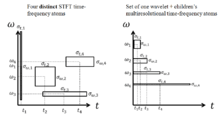STFT time-frequency atoms (left) and DWT time-scale atoms (right). The time-frequency atoms are four different basis functions used for the STFT (i.e. four separate Fourier transforms required). The time-scale atoms of the DWT achieve small temporal widths for high frequencies and good temporal widths for low frequencies with a single transform basis set.

The discrete wavelet transform is less computationally complex, taking O(N) time as compared to O(N log N) for the fast Fourier transform. This computational advantage is not inherent to the transform, but reflects the choice of a logarithmic division of frequency, in contrast to the equally spaced frequency divisions of the FFT (Fast Fourier Transform) which uses the same basis functions as DFT (Discrete Fourier Transform). It is also important to note that this complexity only applies when the filter size has no relation to the signal size. A wavelet without compact support such as the Shannon wavelet would require O(N2). (For instance, a logarithmic Fourier Transform also exists with O(N) complexity, but the original signal must be sampled logarithmically in time, which is only useful for certain types of signals.)

## Definition of a wavelet

There are a number of ways of defining a wavelet (or a wavelet family).

### Scaling filter

An orthogonal wavelet is entirely defined by the scaling filter – a low-pass finite impulse response (FIR) filter of length 2N and sum 1. In biorthogonal wavelets, separate decomposition and reconstruction filters are defined.

For analysis with orthogonal wavelets the high pass filter is calculated as the quadrature mirror filter of the low pass, and reconstruction filters are the time reverse of the decomposition filters.

Daubechies and Symlet wavelets can be defined by the scaling filter.

### Scaling function

Wavelets are defined by the wavelet function ψ(t) (i.e. the mother wavelet) and scaling function φ(t) (also called father wavelet) in the time domain.

The wavelet function is in effect a band-pass filter and scaling it for each level halves its bandwidth. This creates the problem that in order to cover the entire spectrum, an infinite number of levels would be required. The scaling function filters the lowest level of the transform and ensures all the spectrum is covered. See for a detailed explanation.

For a wavelet with compact support, φ(t) can be considered finite in length and is equivalent to the scaling filter g.

Meyer wavelets can be defined by scaling functions

### Wavelet function

The wavelet only has a time domain representation as the wavelet function ψ(t).

For instance, Mexican hat wavelets can be defined by a wavelet function. See a list of a few Continuous wavelets.

## History

The development of wavelets can be linked to several separate trains of thought, starting with Haar's work in the early 20th century. Later work by Dennis Gabor yielded Gabor atoms (1946), which are constructed similarly to wavelets, and applied to similar purposes. Notable contributions to wavelet theory can be attributed to Zweig’s discovery of the continuous wavelet transform in 1975 (originally called the cochlear transform and discovered while studying the reaction of the ear to sound), Pierre Goupillaud, Grossmann and Morlet's formulation of what is now known as the CWT (1982), Jan-Olov Strömberg's early work on discrete wavelets (1983), Daubechies' orthogonal wavelets with compact support (1988), Mallat's multiresolution framework (1989), Akansu's Binomial QMF (1990), Nathalie Delprat's time-frequency interpretation of the CWT (1991), Newland's harmonic wavelet transform (1993) and many others since.

## Wavelet transforms

Main article: Wavelet transform

A wavelet is a mathematical function used to divide a given function or continuous-time signal into different scale components. Usually one can assign a frequency range to each scale component. Each scale component can then be studied with a resolution that matches its scale. A wavelet transform is the representation of a function by wavelets. The wavelets are scaled and translated copies (known as "daughter wavelets") of a finite-length or fast-decaying oscillating waveform (known as the "mother wavelet"). Wavelet transforms have advantages over traditional Fourier transforms for representing functions that have discontinuities and sharp peaks, and for accurately deconstructing and reconstructing finite, non-periodic and/or non-stationary signals.

Wavelet transforms are classified into discrete wavelet transforms (DWTs) and continuous wavelet transforms (CWTs). Note that both DWT and CWT are continuous-time (analog) transforms. They can be used to represent continuous-time (analog) signals. CWTs operate over every possible scale and translation whereas DWTs use a specific subset of scale and translation values or representation grid.

There are a large number of wavelet transforms each suitable for different applications. For a full list see list of wavelet-related transforms but the common ones are listed below:

### Generalized transforms

There are a number of generalized transforms of which the wavelet transform is a special case. For example, Joseph Segman introduced scale into the Heisenberg group, giving rise to a continuous transform space that is a function of time, scale, and frequency. The CWT is a two-dimensional slice through the resulting 3d time-scale-frequency volume.

Another example of a generalized transform is the chirplet transform in which the CWT is also a two dimensional slice through the chirplet transform.

An important application area for generalized transforms involves systems in which high frequency resolution is crucial. For example, darkfield electron optical transforms intermediate between direct and reciprocal space have been widely used in the harmonic analysis of atom clustering, i.e. in the study of crystals and crystal defects. Now that transmission electron microscopes are capable of providing digital images with picometer-scale information on atomic periodicity in nanostructure of all sorts, the range of pattern recognition and strain/metrology applications for intermediate transforms with high frequency resolution (like brushlets and ridgelets) is growing rapidly.

Fractional wavelet transform (FRWT) is a generalization of the classical wavelet transform in the fractional Fourier transform domains. This transform is capable of providing the time- and fractional-domain information simultaneously and representing signals in the time-fractional-frequency plane.

## Applications of wavelet transform

Generally, an approximation to DWT is used for data compression if a signal is already sampled, and the CWT for signal analysis. Thus, DWT approximation is commonly used in engineering and computer science, and the CWT in scientific research.

Like some other transforms, wavelet transforms can be used to transform data, then encode the transformed data, resulting in effective compression. For example, JPEG 2000 is an image compression standard that uses biorthogonal wavelets. This means that although the frame is overcomplete, it is a tight frame (see types of frames of a vector space), and the same frame functions (except for conjugation in the case of complex wavelets) are used for both analysis and synthesis, i.e., in both the forward and inverse transform. For details see wavelet compression.

A related use is for smoothing/denoising data based on wavelet coefficient thresholding, also called wavelet shrinkage. By adaptively thresholding the wavelet coefficients that correspond to undesired frequency components smoothing and/or denoising operations can be performed.

Wavelet transforms are also starting to be used for communication applications. Wavelet OFDM is the basic modulation scheme used in HD-PLC (a power line communications technology developed by Panasonic), and in one of the optional modes included in the IEEE 1901 standard. Wavelet OFDM can achieve deeper notches than traditional FFT OFDM, and wavelet OFDM does not require a guard interval (which usually represents significant overhead in FFT OFDM systems).

### As a representation of a signal

Often, signals can be represented well as a sum of sinusoids. However, consider a non-continuous signal with an abrupt discontinuity; this signal can still be represented as a sum of sinusoids, but requires an infinite number, which is an observation known as Gibbs phenomenon. This, then, requires an infinite number of Fourier coefficients, which is not practical for many applications, such as compression. Wavelets are more useful for describing these signals with discontinuities because of their time-localized behavior (both Fourier and wavelet transforms are frequency-localized, but wavelets have an additional time-localization property). Because of this, many types of signals in practice may be non-sparse in the Fourier domain, but very sparse in the wavelet domain. This is particularly useful in signal reconstruction, especially in the recently popular field of compressed sensing. (Note that the short-time Fourier transform (STFT) is also localized in time and frequency, but there are often problems with the frequency-time resolution trade-off. Wavelets are better signal representations because of multiresolution analysis.)

This motivates why wavelet transforms are now being adopted for a vast number of applications, often replacing the conventional Fourier transform. Many areas of physics have seen this paradigm shift, including molecular dynamics, ab initio calculations, astrophysics, density-matrix localisation, seismology, optics, turbulence and quantum mechanics. This change has also occurred in image processing, EEG, EMG, ECG analyses, brain rhythms, DNA analysis, protein analysis, climatology, human sexual response analysis, general signal processing, speech recognition, acoustics, vibration signals, computer graphics, multifractal analysis, and sparse coding. In computer vision and image processing, the notion of scale space representation and Gaussian derivative operators is regarded as a canonical multi-scale representation.

### Wavelet denoising

Suppose we measure a noisy signal. Assume s has a sparse representation in a certain wavelet bases, andSo.

Most elements in p are 0 or close to 0, andSince W is orthogonal, the estimation problem amounts to recovery of a signal in iid Gaussian noise. As p is sparse, one method is to apply a Gaussian mixture model for p.

Assume a prior,is the variance of "significant" coefficients, andis the variance of "insignificant" coefficients.

Then,is called the shrinkage factor, which depends on the prior variancesand. The effect of the shrinkage factor is that small coefficients are set early to 0, and large coefficients are unaltered.

Small coefficients are mostly noises, and large coefficients contain actual signal.

At last, apply the inverse wavelet transform to obtain## List of wavelets

### Continuous wavelets

#### Complex-valued

1. Ricker, Norman (1953). "WAVELET CONTRACTION, WAVELET EXPANSION, AND THE CONTROL OF SEISMIC RESOLUTION". Geophysics. 18 (4). doi:10.1190/1.1437927.
2. Larson, David R. (2007). "Wavelet Analysis and Applications". Appl. Numer. Harmon. Anal. Birkhäuser: 143–171. |chapter= ignored (help)
3. Mallat, Stephane. "A wavelet tour of signal processing. 1998." 250-252.
4. The Scientist and Engineer's Guide to Digital Signal Processing By Steven W. Smith, Ph.D. chapter 8 equation 8-1: http://www.dspguide.com/ch8/4.htm
5. http://homepages.dias.ie/~ajones/publications/28.pdf
6. http://scienceworld.wolfram.com/biography/Zweig.html Zweig, George Biography on Scienceworld.wolfram.com
7. P. Hirsch, A. Howie, R. Nicholson, D. W. Pashley and M. J. Whelan (1965/1977) Electron microscopy of thin crystals (Butterworths, London/Krieger, Malabar FLA) ISBN 0-88275-376-2
8. P. Fraundorf, J. Wang, E. Mandell and M. Rose (2006) Digital darkfield tableaus, Microscopy and Microanalysis 12:S2, 1010–1011 (cf. arXiv:cond-mat/0403017)
9. M. J. Hÿtch, E. Snoeck and R. Kilaas (1998) Quantitative measurement of displacement and strain fields from HRTEM micrographs, Ultramicroscopy 74:131-146.
10. Martin Rose (2006) Spacing measurements of lattice fringes in HRTEM image using digital darkfield decomposition (M.S. Thesis in Physics, U. Missouri – St. Louis)
11. F. G. Meyer and R. R. Coifman (1997) Applied and Computational Harmonic Analysis 4:147.
12. A. G. Flesia, H. Hel-Or, A. Averbuch, E. J. Candes, R. R. Coifman and D. L. Donoho (2001) Digital implementation of ridgelet packets (Academic Press, New York).
13. J. Shi, N.-T. Zhang, and X.-P. Liu, "A novel fractional wavelet transform and its applications," Sci. China Inf. Sci., vol. 55, no. 6, pp. 1270–1279, June 2012. URL: http://www.springerlink.com/content/q01np2848m388647/
14. A.N. Akansu, W.A. Serdijn and I.W. Selesnick, Emerging applications of wavelets: A review, Physical Communication, Elsevier, vol. 3, issue 1, pp. 1-18, March 2010.
15. Stefano Galli, O. Logvinov (July 2008). "Recent Developments in the Standardization of Power Line Communications within the IEEE". IEEE Communications Magazine. 46 (7): 64–71. doi:10.1109/MCOM.2008.4557044. An overview of P1901 PHY/MAC proposal.
16. J. Rafiee et al. Feature extraction of forearm EMG signals for prosthetics, Expert Systems with Applications 38 (2011) 4058–67.
17. J. Rafiee et al. Female sexual responses using signal processing techniques, The Journal of Sexual Medicine 6 (2009) 3086–96. (pdf)
18. J. Rafiee and Peter W. Tse, Use of autocorrelation in wavelet coefficients for fault diagnosis, Mechanical Systems and Signal Processing 23 (2009) 1554–72.
19. Matlab Toolbox – URL: http://matlab.izmiran.ru/help/toolbox/wavelet/ch06_a32.html
20. Erik Hjelmås (1999-01-21) Gabor Wavelets URL: http://www.ansatt.hig.no/erikh/papers/scia99/node6.html
• Paul S. Addison, The Illustrated Wavelet Transform Handbook, Institute of Physics, 2002, ISBN 0-7503-0692-0
• Ali Akansu and Richard Haddad, Multiresolution Signal Decomposition: Transforms, Subbands, Wavelets, Academic Press, 1992, ISBN 0-12-047140-X
• B. Boashash, editor, "Time-Frequency Signal Analysis and Processing – A Comprehensive Reference", Elsevier Science, Oxford, 2003, ISBN 0-08-044335-4.
• Tony F. Chan and "Jackie (Jianhong) Shen", Image Processing and Analysis – Variational, PDE, Wavelet, and Stochastic Methods, Society of Applied Mathematics, ISBN 0-89871-589-X (2005)
• Ingrid Daubechies, Ten Lectures on Wavelets, Society for Industrial and Applied Mathematics, 1992, ISBN 0-89871-274-2
• Ramazan Gençay, Faruk Selçuk and Brandon Whitcher, An Introduction to Wavelets and Other Filtering Methods in Finance and Economics, Academic Press, 2001, ISBN 0-12-279670-5
• Haar A., Zur Theorie der orthogonalen Funktionensysteme, Mathematische Annalen, 69, pp 331–371, 1910.
• Barbara Burke Hubbard, "The World According to Wavelets: The Story of a Mathematical Technique in the Making", AK Peters Ltd, 1998, ISBN 1-56881-072-5, ISBN 978-1-56881-072-0
• Gerald Kaiser, A Friendly Guide to Wavelets, Birkhauser, 1994, ISBN 0-8176-3711-7
• Stéphane Mallat, "A wavelet tour of signal processing" 2nd Edition, Academic Press, 1999, ISBN 0-12-466606-X
• Donald B. Percival and Andrew T. Walden, Wavelet Methods for Time Series Analysis, Cambridge University Press, 2000, ISBN 0-521-68508-7
• Press, WH; Teukolsky, SA; Vetterling, WT; Flannery, BP (2007), "Section 13.10. Wavelet Transforms", Numerical Recipes: The Art of Scientific Computing (3rd ed.), New York: Cambridge University Press, ISBN 978-0-521-88068-8
• P. P. Vaidyanathan, Multirate Systems and Filter Banks, Prentice Hall, 1993, ISBN 0-13-605718-7
• Mladen Victor Wickerhauser, Adapted Wavelet Analysis From Theory to Software, A K Peters Ltd, 1994, ISBN 1-56881-041-5
• Martin Vetterli and Jelena Kovačević, "Wavelets and Subband Coding", Prentice Hall, 1995, ISBN 0-13-097080-8Look up wavelet in Wiktionary, the free dictionary.Wikimedia Commons has media related to Wavelet.